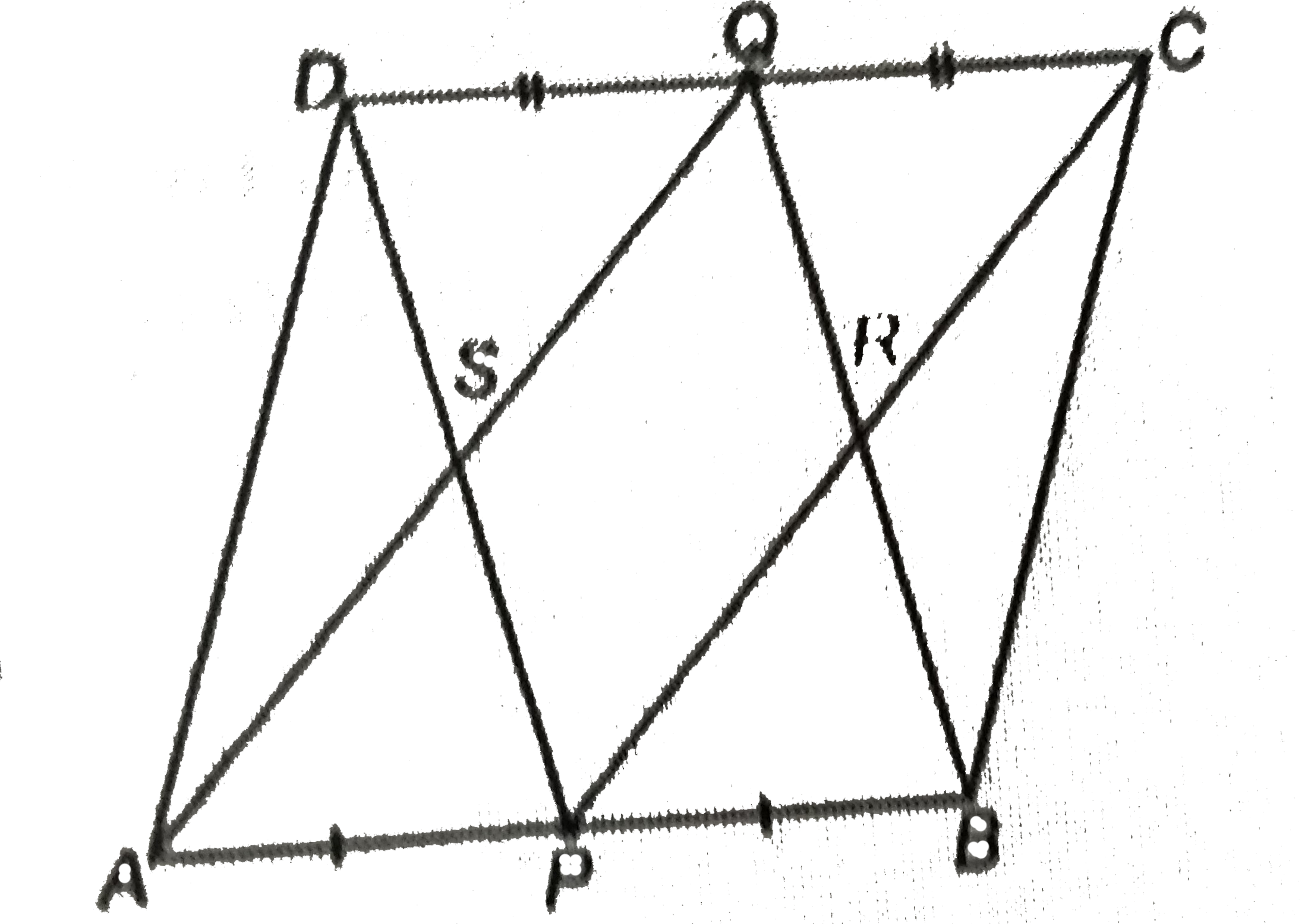# P and Q are the mid-point of the oposite sides AB and CD of a parallelogram ABCD. AQ interects DP at S and BQ interects CP at R. Show that PQRS is a p

42 views

closed
P and Q are the mid-point of the oposite sides AB and CD of a parallelogram ABCD. AQ interects DP at S and BQ interects CP at R. Show that PQRS is a parallelogram.by (97.1k points)
selected by

Given: A parallelogram ABCD in which P and Q are the mid-points of the sides AB and CD respectively. AQ intersects DP at S and BQ interects CP respectively. AQ intersects DP at S and BQ intersects Cp at R.
To prove: PRQS is a parallelogram.
"Proof:"" "DC"||"AB" (because""opposite sides of a parallelogram are parallel")
implies" "AP"||"QC
" "DC"||"AB" "(because"opposite sides of a parallelogram are equal")
implies" "1/2DC=1/2AB
implies" "QC=AP" "(because"P is mid-point of AB and Q is mid-points of CD")
implies" "APCQ"is a parallelogram."" "(becauseAP"||""QCand QC=AP)
therefore" "AQ"||"PC" "(because"opposite sides of a parallelogram are parallel")
implies" "SQ"||"PR"
"Similarly,"" "SP"||"QR
therefore Quadrilateral PQRS is a parallelogram.